NEET  >  Test: Waves - 1

# Test: Waves - 1

Test Description

## 30 Questions MCQ Test | Test: Waves - 1

Test: Waves - 1 for NEET 2023 is part of NEET preparation. The Test: Waves - 1 questions and answers have been prepared according to the NEET exam syllabus.The Test: Waves - 1 MCQs are made for NEET 2023 Exam. Find important definitions, questions, notes, meanings, examples, exercises, MCQs and online tests for Test: Waves - 1 below.
Solutions of Test: Waves - 1 questions in English are available as part of our course for NEET & Test: Waves - 1 solutions in Hindi for NEET course. Download more important topics, notes, lectures and mock test series for NEET Exam by signing up for free. Attempt Test: Waves - 1 | 30 questions in 60 minutes | Mock test for NEET preparation | Free important questions MCQ to study for NEET Exam | Download free PDF with solutions
 1 Crore+ students have signed up on EduRev. Have you?
Test: Waves - 1 - Question 1

### The path difference between two wavefronts emitted by coherent sources of wavelength 5460 Å is 2.1 micron . The phase difference between the wavefronts at that point is _

Test: Waves - 1 - Question 2

### Monochromatic light is that light in which

Test: Waves - 1 - Question 3

### The path difference between two waves  y1= A1 sin wt and y2= A2 cos (wt + f) will be

Detailed Solution for Test: Waves - 1 - Question 3

y1= A1 sin wt
y2= A2 cos (wt + f)
Now, y2 = A2 sin (wt + f + pi/2)
So, phase difference = f + pi/2
Path Difference = (λ/2pi)(f + pi/2)
Hence B.

Test: Waves - 1 - Question 4

The resultant amplitude in interference with two coherent source depends upon _

Detailed Solution for Test: Waves - 1 - Question 4

Two sources are said to be coherent if there always exists a constant phase difference between the waves emitted by these sources. But when the sources are coherent, then the resultant intensity of light at a point will remain constant and so interference fringes will remain stationary.

Test: Waves - 1 - Question 5

The necessary condition for phenomenon of interference to occur is

Detailed Solution for Test: Waves - 1 - Question 5

The necessary condition for phenomenon of interference to occur are:
1. There should be two coherent sources.
2. The frequency and amplitude of both the waves should be same.
3. The propagation of waves should be simultaneously and in same direction.
These are the conditions, no explanation.

Test: Waves - 1 - Question 6

Interference event is observed in

Detailed Solution for Test: Waves - 1 - Question 6

Interference occurs for both transverse and longitudinal waves.
Interference does not indicate whether light is transverse or longitudinal since interference occurs for both types of waves.

Test: Waves - 1 - Question 7

The nature of light which is verified by the interference event is _

Detailed Solution for Test: Waves - 1 - Question 7

The nature of light which is verified by the interference event is wave nature. Even though light has dual nature interference experiments only tell us about the wave nature.

Test: Waves - 1 - Question 8

The phenomenon of interference is based on the principle of _

Detailed Solution for Test: Waves - 1 - Question 8

The phenomenon of interference is based on the principle of superposition.

Test: Waves - 1 - Question 9

The equation for two waves obtained by two light sources are as given below :

y1= A1 sin 3wt, y2 = A2 cos (3wt + p/6). What will be the value of phase difference at the time t _

Detailed Solution for Test: Waves - 1 - Question 9

y1=Asin3 ωt
y2=A2cos(3ωt+p/6)
y2=A2cosd2
y1=A sin(3ωt+p/2- p/2)
=A sin(p/2+3ωt- p/2)
y1=A cos(3ωt- p/2)  [sin(p/2+θ)=Cosθ]
y1=A1cosd1
d2-d1=(3ωt+p/6)- (3ωt- p/2)
=p/6+p/2=2p/3

Test: Waves - 1 - Question 10

Two coherent sources have intensity ratio of 100 : 1, and are used for obtaining the phenomenon of interference. Then the ratio of maximum and minimum intensity will be _

Detailed Solution for Test: Waves - 1 - Question 10

I1/I2 = W1/W2 = 100/1 = (a1/a2)2
a1/a2 = 10/1
a1 = 10x
a2 = x
Imax/lmin = (a1+a2/a1-a2)2
(10x+x/10x-x)2
(11x/9x)2
(11/9)2
121/81

Test: Waves - 1 - Question 11

In coherent sources it is necessary that there

Detailed Solution for Test: Waves - 1 - Question 11

Two sources are said to be coherent if there always exists a constant phase difference between the waves emitted by these sources. But when the sources are coherent, then the resultant intensity of light at a point will remain constant and so interference fringes will remain stationary.

Test: Waves - 1 - Question 12

Two independent monochromatic sodium lamps can not produce interference because

Detailed Solution for Test: Waves - 1 - Question 12

Two sources of light are said to be coherent if they emit light which always has a constant phase difference between them. It means that the two sources must emit radiations of the same wavelength. The two independent sources cannot be coherent because of the fact that independent sources cannot maintain a constant phase difference between them. For experimental purposes, two virtual sources obtained from a single parent source can act as coherent. In such a case, all the random phase changes occurring in the parent source are repeated in the virtual sources also, thus maintaining a constant phase difference between them. Since the wavelength of light waves is extremely small, the two sources must be narrow and must also be close to each other.
Interference can never be obtained with two independent sources of light, such as two bulbs or two candles, due to the fact that any two independent beams of light are always incoherent.

Test: Waves - 1 - Question 13

Figure, shows wave fronts in still water, moving in the direction of the arrow towards the interface PQ between a shallow region and a deep (denser) region. Which of the lines shown may represent one of the wave fronts in the deep region ?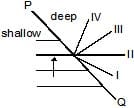Detailed Solution for Test: Waves - 1 - Question 13

The sole reason is 'refraction' in a single word. Refraction of waves involves a change in the direction of waves as they pass from one medium to another. Refraction, or the bending of the path of the waves, is accompanied by a change in speed and wavelength of the waves. Water waves travel fastest when the medium is the deepest. Thus, if water waves are passing from deep water into shallow water, they will slow down. But here it is traveling from shallow water to deep water, then the waves bend in the opposite direction.

Test: Waves - 1 - Question 14

Two coherent monochromatic light beams of intensities I and 4I are superposed. The maximum and minimum possible intensities in the resulting beam are:

Detailed Solution for Test: Waves - 1 - Question 14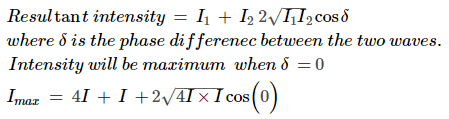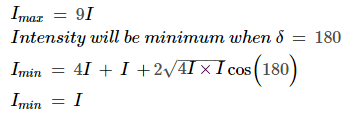Test: Waves - 1 - Question 15

When light is refracted into a denser medium,

Detailed Solution for Test: Waves - 1 - Question 15

Frequency only depends on the source, i.e. frequency changes only when source changes. So, if light ray travels from one medium to another there will be no change in the frequency because the ray still originates from the same source. For any wave,
Lf = c
where, f = frequency, L = wavelength and c = speed of sound
When light travels from one medium to another there will be a change in its velocity. As a result there will be change in its wavelength since there must be no change in the frequency.

Test: Waves - 1 - Question 16

In Young's double slit experiment 62 fringes are visible in the field of view with sodium light (l = 5893Å). If green light (l = 5461Å) is used then the number of visible fringes will be _

Detailed Solution for Test: Waves - 1 - Question 16

Wavelength of light used is inversely proportional to number of fringes observed.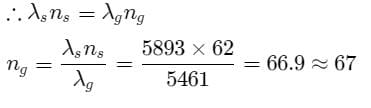Test: Waves - 1 - Question 17

In Young's double slit experiment, the distance of the n-th dark fringe from the centre is _

Detailed Solution for Test: Waves - 1 - Question 17

This is a direct result from NCERT. In Young's double slit experiment, the distance of the nth dark fringe from the centre is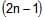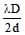Test: Waves - 1 - Question 18

If the yellow light is replaced by the violet light then the interference fringes-

Detailed Solution for Test: Waves - 1 - Question 18

As we know that fringe width is directly proportional to wavelength and since yellow light has higher wavelength than violet light if we change yellow light with violet light then the fringe width will decrease.

Test: Waves - 1 - Question 19

If the path difference between the interfering waves is nl, then the fringes obtained on the screen will be

Detailed Solution for Test: Waves - 1 - Question 19

B because of constructive interference.

Test: Waves - 1 - Question 20

The fringe width in a Young's double slit experiment can be increased. If we decrease

Detailed Solution for Test: Waves - 1 - Question 20

Fringe width = λD/d
where λ us wavelength
D is the distance between slits & screen
d is the distance between slits

Test: Waves - 1 - Question 21

In young's double slit experiment, interference pattern is observed on the screen L distance apart from slits, average distance between adjacent fringes is x and slits separation is d, then the wavelength of light will be _

Detailed Solution for Test: Waves - 1 - Question 21

Fringe width = λD/d
where λ us wavelength
D is the distance between slits & screen
d is the distance between slits
ATQ: x = Lλ/d => λ = xd/L

Test: Waves - 1 - Question 22

Plane microwaves from a transmitter are directed normally towards a plane reflector. A detector moves along the normal to the reflector. Between positions of 14 successive maxima, the detector travels a distance 0.14 m. If the velocity of light is 3 × 108 m/s, find the frequency of the transmitter.

Detailed Solution for Test: Waves - 1 - Question 22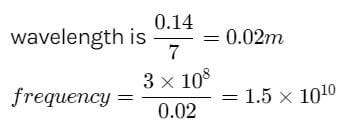Test: Waves - 1 - Question 23

In YDSE how many maxima can be obtained on the screen if wavelength of light used is 200nm and d = 700 nm.

Detailed Solution for Test: Waves - 1 - Question 23

Given that λ = 200nm, d=700nm
θ = λ/d
We know that maximum angle can be 900
No of rings that can be obtained say n,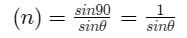If θ is so small then −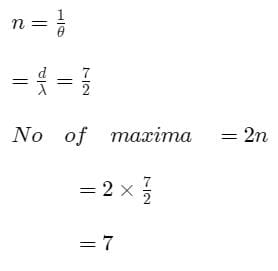Test: Waves - 1 - Question 24

In a YDSE, the central bright fringe can be identified.

Detailed Solution for Test: Waves - 1 - Question 24

If we use white light instead of monochromatic light then we would observe the formation of bright colored fringes forming an interference patterns which resemble the seven colors of a VIBGYOR. Here the white light would split into its fundamental colors and for the central fringe the colors won’t split.

Test: Waves - 1 - Question 25

In Young's double slit experiment, the wavelength of red light is 7800 Å and that of blue light is 5200 Å. The value of n for which nth bright band due to red light coincides with (n + 1)th bright band due to blue light, is

Detailed Solution for Test: Waves - 1 - Question 25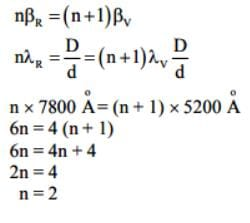Test: Waves - 1 - Question 26

If the Young's double slit experiment is performed with white light, then which of the following is not true

Detailed Solution for Test: Waves - 1 - Question 26

The central fringe will be white because all the component color bands will superimpose there. The option (a) is true.
Since the fringes of different wavelengths of light have different fringe-widths, there will not be a completely dark fringe. Option (b) is true.
Since the wavelength of the violet light is nearly half of the red light, the fringe-width of the violet light is half of the red light. Since the central bright violet fringe combines with other fringes to form the white fringe, the next to it will be a minima for violet light. So violet will not be next to the central white fringe. Next will be a very thin band of white light minus violet light which will give a shade of off white. In this way, the components of indigo, blue, green, yellow and orange colors will be withdrawn respectively in the very thin consecutive bands. These will effect in different off white shades which cannot be clearly differentiated from the white band. The first color away from the center will be recognized as red when in these very thin bands yellow + orange + red, orange + red or red remains. Hence the option (d) is true.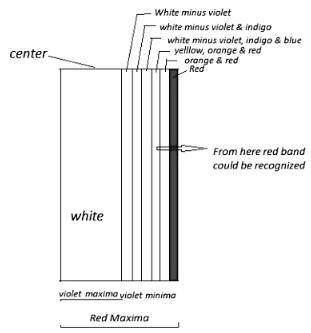Young's Double Slit Experiment with White light

Test: Waves - 1 - Question 27

Two identical narrow slits S1 and S2are illuminated by light of wavelength l from a point source P. If, as shown in the diagram above the light is then allowed to fall on a screen, and if n is a positive integer, the condition for destructive interference at Q is that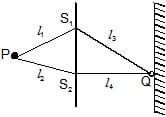Detailed Solution for Test: Waves - 1 - Question 27

Path difference = (l1 + l3) – (l2 + l4)
for destructive interference
Path difference = (2n + 1) λ/2
from (1) and (2)
(l1 + l3) – (l2 + l4) = (2n + 1) λ/2

Test: Waves - 1 - Question 28

In a Young's double slit experiment, a small detector measures an intensity of illumination of I units at the centre of the fringe pattern. If one of the two (identical) slits is now covered, the measured intensity will be

Detailed Solution for Test: Waves - 1 - Question 28

If the intensity of central maxima is I, then intensity due to individual source must be I/4. So, if one of the slit is closed then due to remaining slit, intensity will be I/4.

Test: Waves - 1 - Question 29

In a young double slit experiment D equals the distance of screen and d is the separation between the slit. The distance of the nearest point to the central maximum where the intensity is same as that due to a single slit, is equal to

Detailed Solution for Test: Waves - 1 - Question 29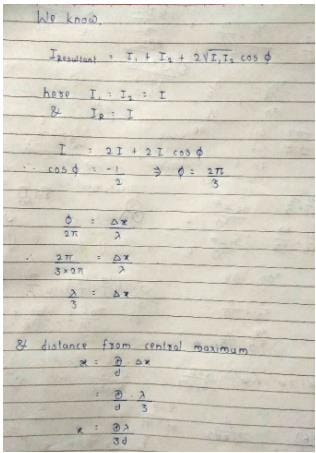Test: Waves - 1 - Question 30

A beam of light consisting of two wavelength 6300 Å and l Å is used to obtain interference fringes in a Young's double slit experiment. If 4th bright fringe of 6300 Å coincides with 5th dark fringe of l Å, the value of l (in Å) is

Detailed Solution for Test: Waves - 1 - Question 30

4th bright fringe(6300A°)
à5th dark fringe (λA°)
For bright fringe
Δz=nλ  (n=0,1,2,3….)
For dark fringe
Δz=(2n-1/2) λ (n= from 1)
4x6300=9/2λ
λ=5600A°

 Use Code STAYHOME200 and get INR 200 additional OFF Use Coupon Code
Information about Test: Waves - 1 Page
In this test you can find the Exam questions for Test: Waves - 1 solved & explained in the simplest way possible. Besides giving Questions and answers for Test: Waves - 1, EduRev gives you an ample number of Online tests for practice

### How to Prepare for NEET

Read our guide to prepare for NEET which is created by Toppers & the best Teachers